cancel
Showing results for
Did you mean:Helper II

## Issue with calculating month-to-month average using AVERAGE or AVERAGEX formula in Power BI

Hi there,

I'm having an issue and we are not able to create a measure to calculate the average of these month-to-month data. We are not sure what is happening when we use the average or averagex formula.

What I am trying to calculate is the sum of a column where we have different prices and then I want to calculate the month-to-month average of each data point, but I am not sure what it is calculating for me.

Measures: 17.1 Provider Cost Sum = SUM('17-Volume billing'[Provider Cost]) Average = AVERAGEX('17-Volume billing',[17.1 Provider Cost Sum])Thanks.

1 ACCEPTED SOLUTIONSuper User

@jesusssss

``````Average =
VAR MaxDate =
MAX ( Calendario[Date] )
VAR MinDate =
CALCULATE ( MIN ( Calendario[Date] ), ALLSELECTED ( Calendario ) )
VAR DatesOnAndBefore =
CALCULATETABLE (
VALUES ( Calendario[Mes] ),
Calendario[Date] <= MaxDate,
Calendario[Date] >= MinDate,
ALLSELECTED ()
)
RETURN
IF (
[17.1 Provider Cost Sum] <> BLANK (),
AVERAGEX ( DatesOnAndBefore, [17.1 Provider Cost Sum] )
)``````
26 REPLIES 26Super User

@jesusssss

``````Average =
VAR CurrentDia =
MAX ( 'Table'[Dia] )
VAR DiaOnAndBefore =
FILTER ( ALLSELECTED ( 'Table'[Dia] ), 'Table'[Dia] <= CurrentDia )
RETURN
IF (
[17.1 Provider Cost Sum] <> BLANK (),
AVERAGEX ( DiaOnAndBefore, [17.1 Provider Cost Sum] )
)``````Helper II

I just tried it, but I'm still getting the same result as with the average measure. What I think is that it has to do with the calendar[date], meaning that when you put Table[day], I thought you were referring to my date calendar table, but it's still not working. Maybe I have to create an additional column in the calendar table?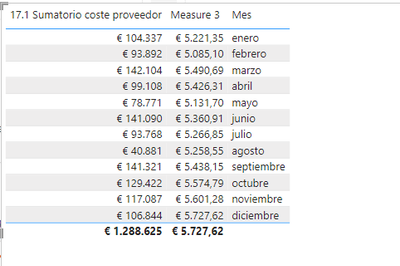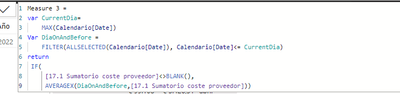Super User

@jesusssss

``````Average =
VAR CurrentDate =
MAX ( Calendario[Date] )
VAR DatesOnAndBefore =
CALCULATETABLE (
VALUES ( Calendario[Mes] ),
Calendario[Date] <= CurrentDate,
ALLSELECTED ()
)
RETURN
IF (
[17.1 Provider Cost Sum] <> BLANK (),
AVERAGEX ( DatesOnAndBefore, [17.1 Provider Cost Sum] )
)``````Helper II

Hi, I have already tested it and the result is this. They are giving me the same data. I don't know if you know why this could be happening, if it could be an error in the calculation of the supplier cost sum column or in the calendar.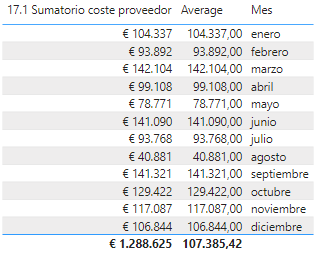thanks for the help,Let's see if we can figure out what the error is.Super User

@jesusssss
Anything else in the filter context other than Month? Also lets avoid using the [Date].[Month] and create the column [Month] or [Year-Month] in the Calendar table.Helper II

The only filters that exist are the year and month filters.I just created a column in my calendar table that refers to the months.But the average is still coming out wrongSuper User

@jesusssss
In the table and slicer also use the [monthname] column. Do the same for the [Year]Helper II

I have changed the filters on the slicers for the new dates columns of year and month that i have alredy created but it doesn´t work.Super User

@jesusssssPlease paste the final DAX you're usingHelper II

You are right, that section was not changed but I changed it and it seems that it takes me new data but it still does not average the excelSuper User

@jesusssss

``````Average =
VAR MaxDate =
MAX ( Calendario[Date] )
VAR MinDate =
CALCULATE ( MIN ( Calendario[Date] ), ALLSELECTED ( Calendario ) )
VAR DatesOnAndBefore =
CALCULATETABLE (
VALUES ( Calendario[Mes] ),
Calendario[Date] <= MaxDate,
Calendario[Date] >= MinDate,
ALLSELECTED ()
)
RETURN
IF (
[17.1 Provider Cost Sum] <> BLANK (),
AVERAGEX ( DatesOnAndBefore, [17.1 Provider Cost Sum] )
)``````Helper II

I already tested it and it works correctly, thank you very very much. The only thing is that the month column I created appears to be disordered, I don't know if you know how to fix it.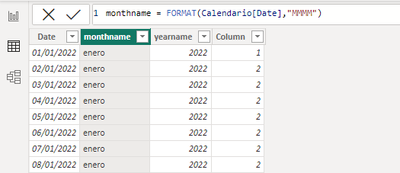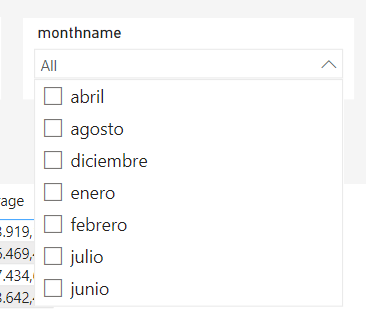Super User

Create a month number column and use "sort by column " to sort the monthname by monthnunberHelper II

Hi

Yesterday, I thought I had managed to sort it out, but apparently not because when I sort it by the 'date' column with the formula I created, it messes up the average again.

This is without sorting.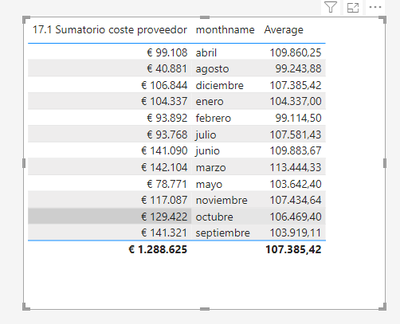And this is when I sort it

This is the columns that I have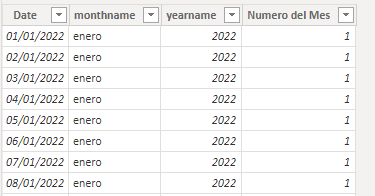Calendario = CALENDAR(DATE(2022,1,1),MAXX(Fecha,Fecha[FechaEntregaPrevista]))

monthname = FORMAT(Calendario[Date],"MMMM")

yearname = YEAR(Calendario[Date])

Number of the month = MONTH(Calendario[Date])

I don't know what's going on, whether it's a problem with the calendar table or not. I'm not sure if there is another way to sort the column or to create a 'month' column.

Thanks.Super User

Hi @jesusssss
Sorry I did not notice your reply. You need to use "sort by column" to sort the [monthname] column by the [Number of the month] columnHelper II

hi @tamerj1

But when I do what you tell me, the data is duplicated in the summation and in the average, unlike putting the average without sorting which appears correctly to me."

Before sortafter sort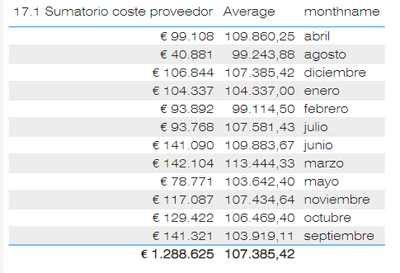Thanks for the help.Super User

@jesusssss
Double check the Date format in both 'Fecha' and 'Calendario' tables interms of "DD/MM/YYYY" or  "MM/DD/YYYY"Helper II

Hi @tamerj1

This are all the formats: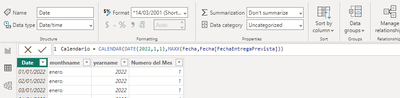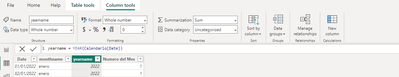Its something wrong here?

ThanksSuper User

Hi @jesusssss
Can we connect?Helper II

hi @tamerj1

Of course, through which platform would you like to connect?Announcements#### Exclusive opportunity for Women!

Join us for a free, hands-on Microsoft workshop led by women trainers for women where you will learn how to build a Dashboard in a Day!#### Power Platform Conference-Power BI and Fabric Sessions

Join us Oct 1 - 6 in Las Vegas for the Microsoft Power Platform Conference.Top Solution Authors
Top Kudoed Authors
Users online (1,822)